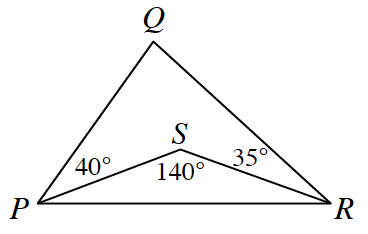### Home > GC > Chapter 12 > Lesson 12.2.1 > Problem12-56

12-56.In $\Delta PQR$ at right, what is $m\angle Q$? Explain how you found your answer.

There are multiple ways to find the measure of $\angle Q$.

Examine the quadrilateral $PQRS$. The sum of the interior angles should be $360^\circ$.

Check your answer! Is your answer for $m\angle Q$ $\Delta PQR$ and $\Delta PSR$ possible?